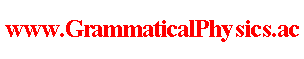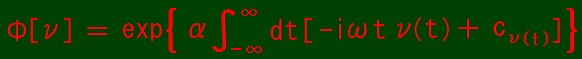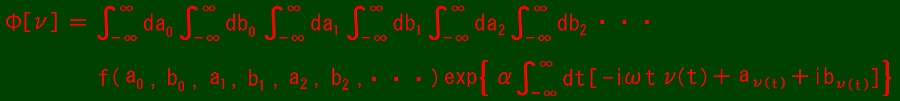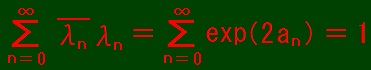since 2006 Help　Sitemap < Forum > < Products > < Quantum History Theory > < Exact Solution of Uda Equation (5) > In this page, I write exact solutions of Energy Representation of Uda Equation (1). Any unentangled solution is functional Φ such thatwhere ∀n ∈ Z; cn ∈ C. The quantum history represented by this solution is the same history as represented by wave function ψ such that ψ(n, t) ＝ exp(-iωtn + cn) ＝ λn exp(-iωnt) in the old grammar. Here, λn ≡ exp(cn). If A†A|n＞ ＝ n|n＞, Σn=0∞ ψ(n, t)|n＞ ＝ Σn=0∞ λnexp(-iωnt)|n＞, Σn=0∞ ψ(n, 0)|n＞ ＝ Σn=0∞ λn|n＞. Of course, any superposition of unentangled solutions is also a solution. Moreover, any entangled solution perhaps is a superposition of unentangled solutions. It is functional Φ such thatwhere ∀n ∈ Z; an ∈ R and bn ∈ R and f(a0, b0, a1, b1, a2, b2, ・・・) ∈ C. cn = an + ibn, λn = exp(an + ibn). Normalization condition for initial state is that. To let the integral converge, b and ν is restricted. However, I do not put such a condition because there is no ambiguity even if the integral diverges. --- The content of this page was developed in Number Representation of Uda Equation @ Quantum History Theory @ Problems. Author Yuichi Uda, Write start at 2019/11/08/17:22JST, Last edit at 2019/11/22/18:45JST